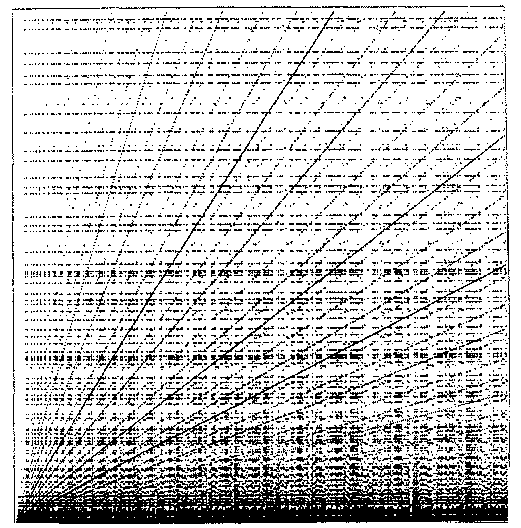# Cliff Pickover's Patterns in the Mysterious Hailstone (3n+1) NumbersA particularly famous problem in number theory, the hailstone problem, has fascinated mathematicians for several decades. (I love making graphics showing patterns in these numbers.) The problem has been studied primarily because it is so simple to state yet apparently intractably hard to solve. This problem is also known as the 3n+1 problem, the Collatz algorithm, and the Syracuse problem. The hailstone algorithm is defined by the function h: N -> N on the set of positive integers: h(n) = n/2 if n even or 3n + 1 if n odd where n is any initial positive integer. Let k(n) be the kth iterate of h(n) . A sequence of hailstone numbers is generated in a mathematical feedback loop where hk(n) = h(hk-1(n)) for k memberof N and can be represented as H(n) = {hk (n) } k = 1,2,3, ....

For example, the hailstone sequence for 3 is: H(3) = { 3, 10, 5, 16, 8, 4, 2, 1, 4, ...}.

Below is H(n) vs. n. This is a plot for a range of starting positive integers (1 < n < 1000) on the x-axis. The y-axis is cut off at H(n)=1000.All the strange plots in my books, such as this one, reveal a pattern of diagonal lines of varying density which pass through the origin, a pattern of horizontal lines (visually reminiscent of preferred energy state diagrams in quantum mechanics) and a diffuse "background" of chaotically positioned dots. An interpretation of the existence of the horizontal lines is immediate: they represent certain values which are much commoner than others and far too common to be explained by any statistical process. Other mathematicians have noted preferred states in the hailstone numbers (Hayes, 1984), and an outstanding example is state 9,232. Of the first 1,000 integers more than 350 have their maximum at 9,232.

The existence of the pronounced diagonal lines is probably indicative of "likely" transformations which the 3n + 1 sequence naturally gives rise to. For the hailstones, we are often multiplying by 3 and then dividing by 2. Therefore, the linear transformation y = (3/2)x is quite common (we can eliminate the + 1 in n+1 for large x).

Like hailstones falling from the sky through storm clouds, this sequence drifts down and up, sometimes in seemingly haphazard patterns. Also like hailstones, hailstone numbers always seem eventually to fall back down to the ground (the integer 1). In fact, it is conjectured that every hailstone sequence ends in the cycle 4,2,1,4,... This hailstone conjecture has been numerically checked for a large range of n, and the current record has been set by N. Yoneda who has checked all integers less than 2 40~ 1.2 * 1012 (see Legarias (1985)).

High-speed computers have allowed investigators to check the validity of many mathematical conjectures, and various mathematical evidence now goes into unimaginably large numbers. However, numerical evidence should be viewed with caution and is sometimes inadequate. For example, consider the fact that integral from 2 to x of 1/ln t dt - pi (x) is positive for all x <= 1012 and probably far beyond (pi(x) is the number of prime numbers less than or equal to x) (Wagon, 1985)). This massive amount of evidence previously led researchers astray; however, it is now known that a sign change does occur, but all that is currently known is that the first sign change occurs below 1.65 * 101165 . A variety of cash awards have been offered for a solution to the hailstone problem.

A number of researchers have noted that the hailstone sequence gives rise to a mixture of regularity and disorder: it is definitely not random, but the pattern resists interpretation. This problem in number theory can be placed in a much larger context of chaos theory which involves the study of a range of mathematical and physical phenomena exhibiting a sensitive and often irregular dependence on initial conditions. Computer graphics is a good method by which patterns in this hailstone sequence can be made more obvious to the mathematician. Unfortunately, computer graphics has been little exploited in 3n+1 work .

Watching the figures evolve dynamically upon the screen helps to give an appreciation of the complicated behavior of 3n+1 numbers. The H(n) maps clearly display preferred states, but exactly why these states and clusters of states exist is unclear. Every possible integer state and trajectory length (path before returning to 1) can be produced -- but again some numbers appear more often than others. As Paul Erdos commented on the complexity of 3n+1 numbers, "Mathematics is not yet ready for such problems."

For more information on hailstone numbers and these hailstone number plots computed for larger ranges, see: Clifford Pickover, Computers, Pattern, Chaos and Beauty and Wonders of Numbers.

Other writers and mathematicians have looked at this problem: Legarias (1985), Wagon (1985), Garner (1981), Crandall (1978), Hayes (1984).